# Point-Slope Form

Point-Slope Form

In this chapter, you will learn about the point slope form equation, the slope of a line equation, the point-slope formula, and point-slope examples. Check-out the interactive simulations to know more about the lesson and try your hand at solving a few interesting practice questions on Point-Slope Form at the end of the page.

Now, that we're done with the formalities, here's a bit of info for you. Did you know that the equation of a line is an equation that is satisfied by each and every point on the line? This means that a linear equation in two variables represents a line.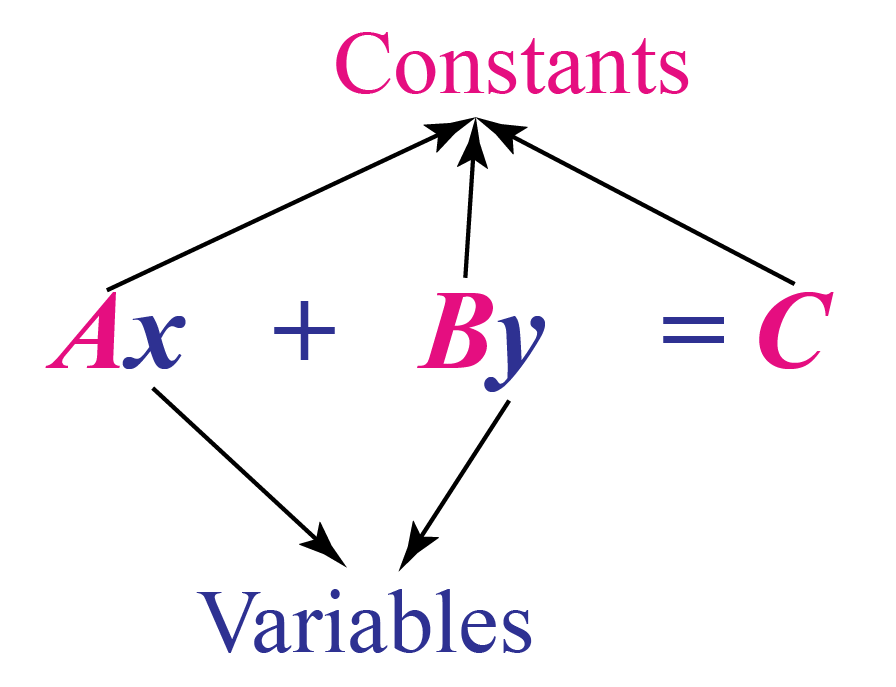The equation of a line can be found through various methods depending on the available information.

Some of the methods are:

• Point-slope form
• Slope-intercept form
• Intercept form
• Two-point form

We will study the point-slope form on this page in detail.

## Lesson Plan

 1 What is Point-Slope Form? 2 Important Notes on Point-Slope Form 3 Solved Examples on Point-Slope Form 4 Thinking Out of the Box 5 Interactive Questions on Point-Slope Form

## What is Point-Slope Form?

The formula of point-slope form is used to find the equation of a line.

This formula is used only when we know the slope of the line and a point on the line.

The equation of a line whose slope is $$m$$ and which passes through a point $$(x_1, y_1$$) is found using the point-slope form.

The equation of the point-slope form is:

 $${y-y_1=m(x-x_1)}$$

where $$(x, y)$$ is a random point on the line.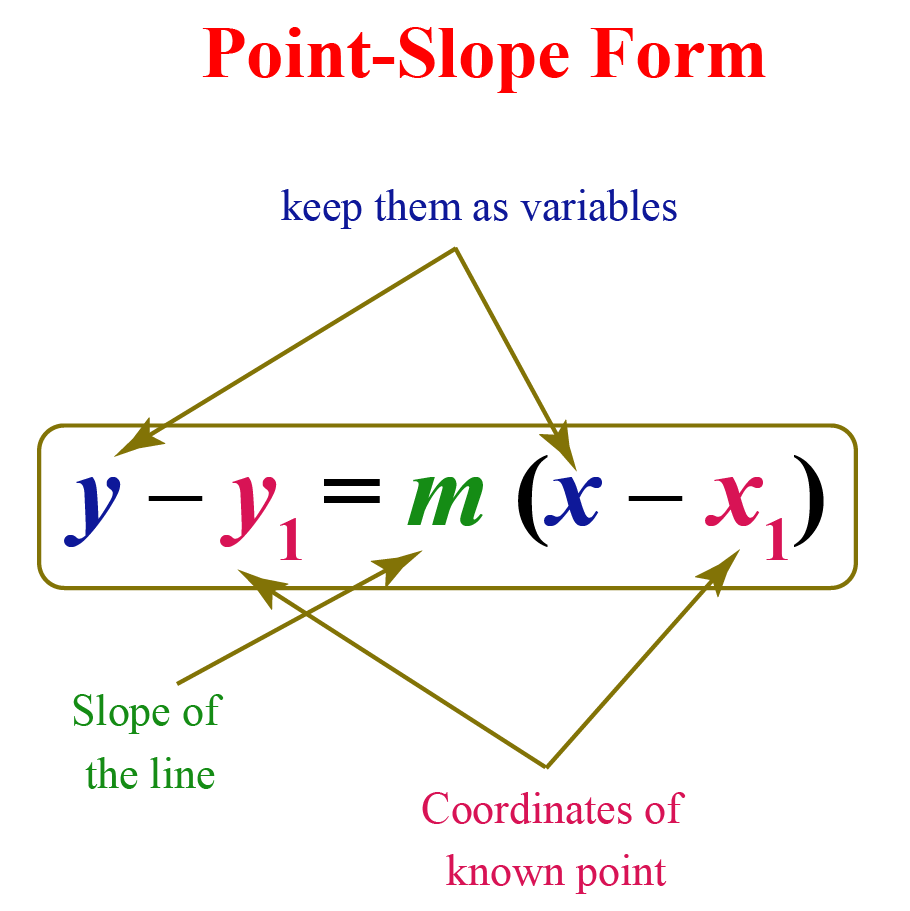## How to Find Point-Slope Form?

Let us see how to find the point-slope form (i.e. the proof of the formula of point-slope form).

We will derive this formula using the equation for the slope of a line.

Let us consider a line whose slope is $$m$$.

Let us assume that $$(x_1, y_1$$) is a known point on the line.

Let $$(x,y)$$ be any other random point on the line whose coordinates are not known.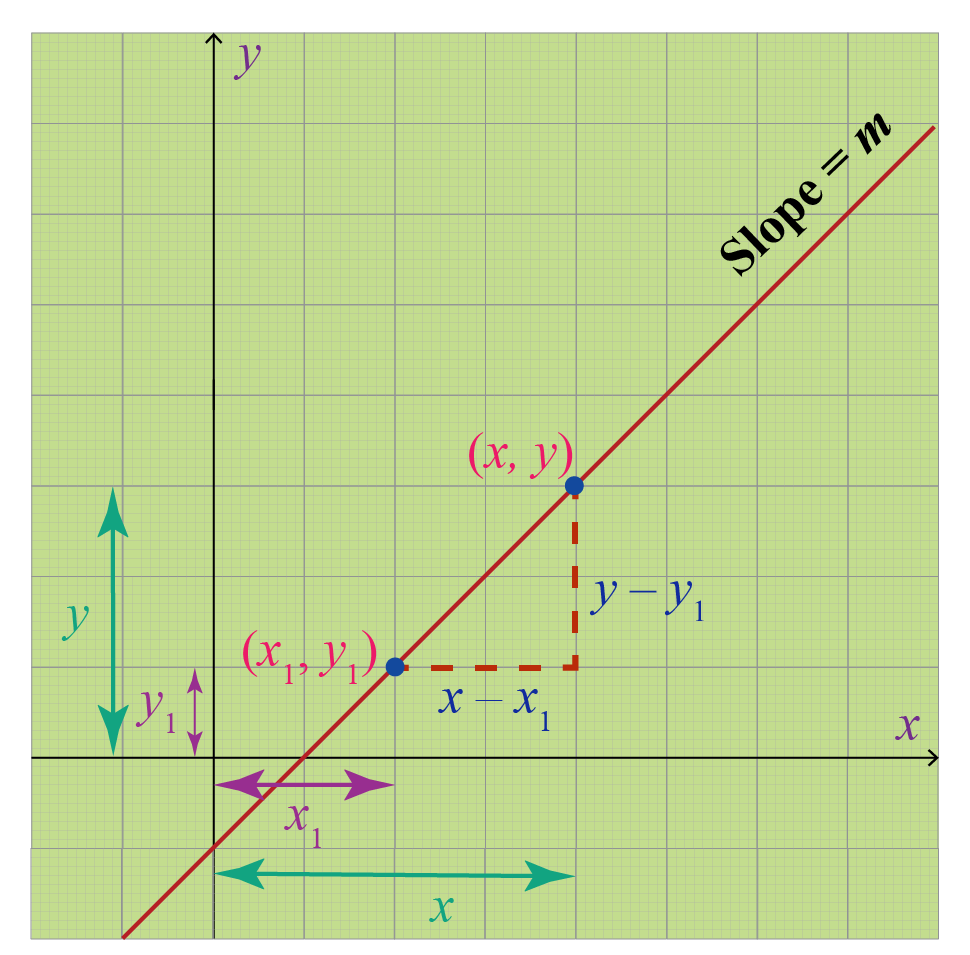We know that the equation for the slope of a line is:

\begin{aligned}\text{Slope }&= \dfrac{ \text{Difference in y-coordinates}}{ \text{Difference in x-coordinates}}\\[0.2cm]m&= \dfrac{y-y_1}{x-x_1} \end{aligned}

Multiplying both sides by $$(x-x_1)$$,

$m(x-x_1) = y-y_1$

This can be written as,

$y-y_1=m(x-x_1)$

Hence the point-slope formula is proved.

## Examples of Point-Slope Form

Some examples of the point-slope form are shown here.

1. The equation of a line with slope $$-1$$ and a point $$(1, 2$$) is found using: $y-2=-1(x-1)$
2. The equation of a line with slope $$\dfrac{3}{2}$$ and a point $$\left( \dfrac{-1}{2}, \dfrac{2}{3} \right)$$ is found using: $y- \dfrac{2}{3}= \dfrac{3}{2} \left(x- \left(- \dfrac{1}{2} \right) \right)$
3. The equation of a line with slope $$0$$ and a point $$(3, -2$$) is found using: $y-(-2) =0(x-3)$

In each of these cases, we can simplify the equation further and get it to the form $$y=mx+b$$.

## Point-Slope Form Calculator

Here is the point-slope form calculator.

Here, you can find the point-slope form of a line by changing the values of $$m, x_1$$, and $$y_1$$

Also, you can see how the graph changes accordingly.

 Example 4

Find the equation of a line that passes through two points $$(1, 3)$$ and $$(-2, 4)$$ using the point-slope form and express the answer in the standard form.

Solution:

The given two points are:

\begin{aligned} (x_1, y_1)&=(1,3)\\[0.2cm] (x_2,y_2)&=(-2, 4) \end{aligned}

To use the point-slope form, we first need to find the slope of the line.

The slope of the given line is found using:

\begin{aligned} m &= \dfrac{y_2-y_1}{x_2-x_1}\\[0.2cm]&= \dfrac{4-3}{-2-1}\\[0.2cm]&= \dfrac{1}{-3}\\[0.2cm]&=- \dfrac{1}{3} \end{aligned} The equation of the line is found using the point-slope form. \begin{aligned} y-y_1&=m(x-x_1)\\[0.2cm]y-3&=- \dfrac{1}{3}(x-1)\\[0.2cm] y-3&=- \dfrac{1}{3} x+ \dfrac{1}{3}\\[0.2cm] \text{Adding 3 }& \text{ on both sides},\\[0.2cm] y&= - \dfrac{1}{3}x+ \dfrac{10}{3} \end{aligned}

To express this in the standard form, first we multiply both sides by $$3$$

$3y=-x+10$

Adding $$x$$ on both sides,

$x+3y=10$

Thus, the equation of the given line in the standard form is:

 $$\therefore$$ $${x+3y=10}$$Think Tank
1. Which of the following graphs may represent the equation $$\mathbf{y+2= -2(x-1)}$$?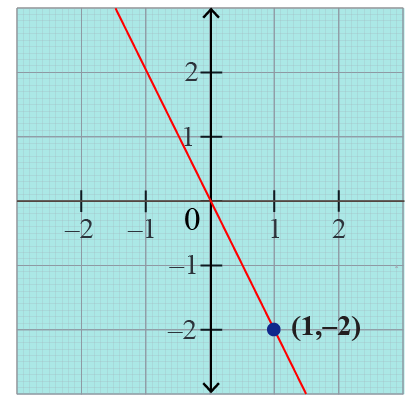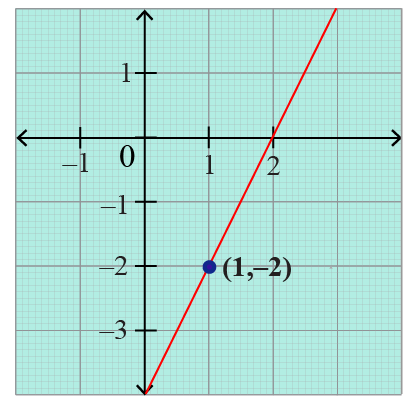## Interactive Questions

Here are a few activities for you to practice. Select/type your answer and click the "Check Answer" button to see the result.

## Let's Summarize

We hope you enjoyed learning about Point-Slope Form with the simulations and practice questions. Now you will be able to easily solve problems on Point Slope form equation, slope of a line equation and also how to find point-slope form.

## About Cuemath

At Cuemath, our team of math experts is dedicated to making learning fun for our favorite readers, the students!

Through an interactive and engaging learning-teaching-learning approach, the teachers explore all angles of a topic.

Be it worksheets, online classes, doubt sessions, or any other form of relation, it’s the logical thinking and smart learning approach that we at Cuemath believe in.

## 1. How do you find the point-slope form with a point-slope?

The point-slope formula is a formula that is used to find the equation of a line.

This formula is used only when we know the slope of a line and a point on the line.

The equation of a line whose slope is m and which passes through a point $$(x_1, y_1$$) is found using the point-slope formula.

The equation of the point-slope form is:

 $$\mathbf{y-y_1=m(x-x_1)}$$

Here $$(x, y)$$ is a random point on the line.

## 2. How do you change point-slope form into slope-intercept form?

The point-slope form is of the form:

$y-y_1=m(x-x_1)$

We will solve this equation for $$y$$ which gives an equation of the form $y=mx+b$

This is called the slope-intercept form.

Here is an example.

Example:

The equation of a line with slope -1 and a point $$(1, 2$$) is found using: $y-2=-1(x-1)$

For changing this into the slope-intercept form, we will solve it for $$y$$.

\begin{align} y-2&=-x+1\\[0.2cm] y&= -x+1+2\\[0.2cm] y&=-x+3 \end{align}

## 3. How do you find the point-slope form of a graph?

To find the point-slope form of a line, we just find the slope and a point on the line.

A point on the line can be easily found by looking at its graph.

The slope of a line is found by first finding any two points on the line from its graph and then applying the formula:

$\text{Slope }= \dfrac{ \text{Difference in y-coordinates}}{ \text{Difference in x-coordinates}}$

Download Coordinate Geometry Worksheets
Coordinate Geometry
Grade 9 | Questions Set 1
Coordinate Geometry
Grade 9 | Answers Set 1
Coordinate Geometry
Grade 10 | Questions Set 1
Coordinate Geometry
Grade 10 | Answers Set 1
More Important Topics
Numbers
Algebra
Geometry
Measurement
Money
Data
Trigonometry
Calculus